# Pump Flow

Most aspects of a pump's performance can be associated with the flow of fluid through the pump. Understanding pump performance involves a basic knowledge of pump specifications and pump performance curves.

## Pump Parameters

Pump operation and performance can best be described by a few fundamental parameters; flow rate, pressure, head, power, and efficiency.

• Volume flow rate (Q), also referred to as capacity, is the volume of liquid that travels through the pump in a given time (measured in gallons per minute or gpm). It defines the rate at which a pump can push fluid through the system. In some cases, the mass flow rate (ṁ) is also used, which describes the mass through the pump over time. The volume flow rate is related to mass flow rate by the fluid density (ρ) via the equation: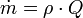When selecting pumps, the flow rate or rated capacity of the pump must be matched to the flow rate required by the application or system.

• Pressure is a measure of resistance: the force per unit area of resistance in the system. The pressure rating of a pump defines how much resistance it can handle or overcome. It is usually given in bar or psi (pounds per square inch). Pressure, in conjunction with flow rate and power, is used to describe pump performance. Centrifugal pumps, however, typically use head (described below) instead of pressure to define the energy or resistance of the pump, since pressure in a centrifugal pump varies with the pumped fluid's specific gravity.

When selecting pumps, the rated operating or discharge pressure of the pump must be equal to or more than the required pressure for the system at the desired flow rate.

• Head is the height above the suction inlet that a pump can lift a fluid. It is a shortcut measurement of system resistance (pressure) which is independent of the fluid's specific gravity. It is defined as the mechanical energy of the flow per unit weight. It is expressed as a column height of water given in feet (ft) or meters (m). In other words, if water was pumped straight up, the pump head is equivalent to the height it reaches.

Pump head (H) can be converted to pressure (P) using the specific gravity (SG) of the fluid by the equation:

P=0.434 • H • (SG)

or by the density of the fluid (ρ) and the acceleration due to gravity (g):

P=H • ρ • g

When selecting centrifugal pumps, the rated pump head must be equal to or greater than the total head of the system (total dynamic head or TDH) at the desired flow rate.

Selection Tip: Pump head in a centrifugal pump will be the same for all liquids if the shaft is spinning at the same speed. The only difference between fluids is the amount of power needed to get the shaft to the proper speed (rpm). The higher the fluid's specific gravity (SG), the more power is required.

Another specification to consider is net positive suction head (NPSH) - the difference between the pump's inlet stagnation pressure head and the vapor pressure head. The required NPSH is an important parameter in preventing cavitation in a pump. Cavitation happens inside a pump when the local pressure falls below the vapor pressure of the liquid being pumped, causing the liquid to boil.

Selection Tip: The pressure inside the pump should be above the NPSH to avoid cavitation, which can result in noise, vibration, reduced efficiency, and damage to impeller blades.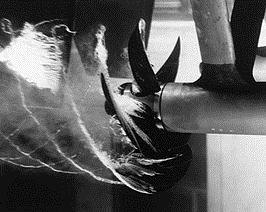Boat propeller cavitation. In the confines of a pump during operation, cavitation to an impeller can be much more devastating.

• Power: Net head is proportional to the power actually delivered to the fluid, called output power (Pout) or the water horsepower (measured in horsepower or hp). This is the horsepower rating which describes the useful work the pump will do to the fluid. It can be calculated by the equation:

Pout=ṁgH=ρgQH

where:
ρ is fluid density
g is the acceleration due to gravity
Q is the volumetric flow rate
ṁ is the mass flow rate

In all pumps there are losses due to friction, internal leakage, flow separation, etc. Because of these losses, the external power supplied to the pump, called the input power (Pin) or brake horsepower, is always larger than the water horsepower. This specification is typically provided by the pump manufacturer as a rating or in the pump's performance curve and is used to select the proper motor or power source for the pump.

Selection Tip: When determining the required power from a typical pump performance curve (discussed below), it is best to use the values at the end of the curve to ensure adequate supply at most operating conditions. For operations with little system variation (e.g. refineries), use the value at the operating point plus 10%.

• Pump efficiencypump) defines the percentage of energy supplied to the pump that is converted into useful work. It is the ratio between the water horsepower and brake horsepower (useful power vs. required power):

ηpump=Pout/Pin

Keep in mind that any efficiency rating of the pump given by the manufacturer assumes certain system conditions such as the type of fluid transported: water is a typical standard. The efficiency may not be accurate if these assumptions differ from the consumer's intended application.

Selection Tip: A more efficient pump is not always the best choice when considering energy costs. For example, a pump that runs at 40% efficiency would be a better choice than one in the same family which is 60% efficient but requires twice the power.

## Pump Performance Curves

All pumps have a characteristic or performance curve that describes the flow rate produced at net or total head. Pump specifications relating head and flow rate correlate to those found on its characteristic curve. A simplified curve for a centrifugal pump will look something like this: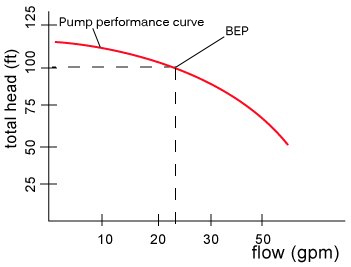Original Image Credit: Pumpfundamentals.com

The pump curve illustrates the available total head at a given flow rate of the pump. Generally, more head is available in the pump as flow rate decreases. Manufacturers usually designate an optimum or best efficiency point (BEP) of the curve, which is indicated in this graph by the dotted line. Thus, this pump runs best when supplying a net head of 100 ft, which will provide a flow rate of 23 gpm.

When selecting a pump for incorporation into a system, users should map the system curve alongside the pump curve. A simplified incorporation of this curve may look something like this: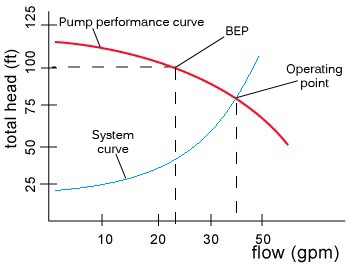The system curve illustrates the required head for different flow rates in the system. It is constructed using a form of Bernoulli's equation for fluid mechanics, which is beyond the scope of this guide. Generally, more head is required as flow rate increases due to frictional forces and other losses in the system. The operating point of the pump in a system should be where the pump curve and system curve intersect. The best pump choice for a system is one in which the required operating point intersects at the pump’s BEP.

Selection Tip: Since every system is unique and has specific head requirements, the ideal choice mentioned above is not always commercially available.

Positive displacement pumps do not utilize fluid momentum, meaning that flow rate is relatively independent of pump head. Thus, (unlike dynamic pumps) positive displacement pumps have a definite capacity across a wide range of head pressures (as shown in the characteristic curve below). Slippage is the result of high discharge pressures causing some liquid to leak back to the pump suction, reducing capacity.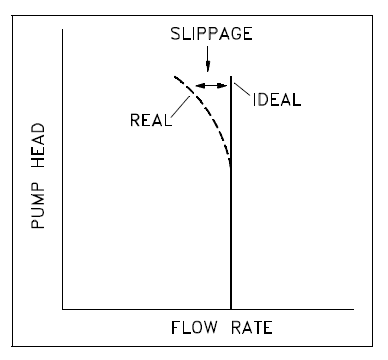Image Credit: ITU.edu

Pump failure results when the total head of the system exceeds the maximum head of the pump.